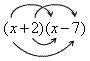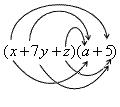# Multiplying Polynomials

To multiply two polynomials, you apply the distributive property . Consider a simple example: multiplying a monomial $3x$ by the binomial $x-2$ .

$\begin{array}{l}3x\cdot \left(x-2\right)=\left(3x\right)\cdot \left(x\right)+\left(3x\right)\cdot \left(-2\right)\\ \text{\hspace{0.17em}}\text{\hspace{0.17em}}\text{\hspace{0.17em}}\text{\hspace{0.17em}}\text{\hspace{0.17em}}\text{\hspace{0.17em}}\text{\hspace{0.17em}}\text{\hspace{0.17em}}\text{\hspace{0.17em}}\text{\hspace{0.17em}}\text{\hspace{0.17em}}\text{\hspace{0.17em}}\text{\hspace{0.17em}}\text{\hspace{0.17em}}\text{\hspace{0.17em}}\text{\hspace{0.17em}}\text{\hspace{0.17em}}\text{\hspace{0.17em}}\text{\hspace{0.17em}}\text{\hspace{0.17em}}\text{\hspace{0.17em}}\text{\hspace{0.17em}}\text{\hspace{0.17em}}=3{x}^{2}-6x\end{array}$

When you multiply one binomial by another binomial, you have to use the distributive property repeatedly.

$\begin{array}{l}\left(x+2\right)\cdot \left(x-7\right)=\left(x\right)\left(x-7\right)+\left(2\right)\left(x-7\right)\\ \text{\hspace{0.17em}}\text{\hspace{0.17em}}\text{\hspace{0.17em}}\text{\hspace{0.17em}}\text{\hspace{0.17em}}\text{\hspace{0.17em}}\text{\hspace{0.17em}}\text{\hspace{0.17em}}\text{\hspace{0.17em}}\text{\hspace{0.17em}}\text{\hspace{0.17em}}\text{\hspace{0.17em}}\text{\hspace{0.17em}}\text{\hspace{0.17em}}\text{\hspace{0.17em}}\text{\hspace{0.17em}}\text{\hspace{0.17em}}\text{\hspace{0.17em}}\text{\hspace{0.17em}}\text{\hspace{0.17em}}\text{\hspace{0.17em}}\text{\hspace{0.17em}}\text{\hspace{0.17em}}\text{\hspace{0.17em}}\text{\hspace{0.17em}}\text{\hspace{0.17em}}\text{\hspace{0.17em}}\text{\hspace{0.17em}}\text{\hspace{0.17em}}\text{\hspace{0.17em}}\text{\hspace{0.17em}}=\left(x\right)\left(x\right)+\left(x\right)\left(-7\right)+\left(2\right)\left(x\right)+\left(2\right)\left(-7\right)\end{array}$

There's a shortcut you can use here, known as the " FOIL " method (that stands for F irst, O uter, I nner, L ast.). The product of two binomials is the sum of four simpler products.The product of the F irst terms is: $\left(x\right)\left(x\right)={x}^{2}$

The product of the O uter terms is: $\left(x\right)\left(-7\right)=-7x$

The product of the I nner terms is: $\left(2\right)\left(x\right)=2x$

And the product of the L ast terms is: $\left(2\right)\left(-7\right)=-14$

$\begin{array}{l}\left(x+2\right)\cdot \left(x-7\right)={x}^{2}+\left(-7x\right)+2x+\left(-14\right)\\ \text{\hspace{0.17em}}\text{\hspace{0.17em}}\text{\hspace{0.17em}}\text{\hspace{0.17em}}\text{\hspace{0.17em}}\text{\hspace{0.17em}}\text{\hspace{0.17em}}\text{\hspace{0.17em}}\text{\hspace{0.17em}}\text{\hspace{0.17em}}\text{\hspace{0.17em}}\text{\hspace{0.17em}}\text{\hspace{0.17em}}\text{\hspace{0.17em}}\text{\hspace{0.17em}}\text{\hspace{0.17em}}\text{\hspace{0.17em}}\text{\hspace{0.17em}}\text{\hspace{0.17em}}\text{\hspace{0.17em}}\text{\hspace{0.17em}}\text{\hspace{0.17em}}\text{\hspace{0.17em}}\text{\hspace{0.17em}}\text{\hspace{0.17em}}\text{\hspace{0.17em}}\text{\hspace{0.17em}}\text{\hspace{0.17em}}\text{\hspace{0.17em}}\text{\hspace{0.17em}}\text{\hspace{0.17em}}={x}^{2}-5x-14\end{array}$

You can use a similar strategy to multiply trinomials or other polynomials. For example, to multiply:you need to find six products:

• $\left(x\right)\left(a\right)$
• $\left(7y\right)\left(a\right)$
• $\left(z\right)\left(a\right)$
• $\left(x\right)\left(5\right)$
• $\left(7y\right)\left(5\right)$
• $\left(z\right)\left(5\right)$

and then add them all up to get $ax+7ay+az+5x+35y+5z$ .